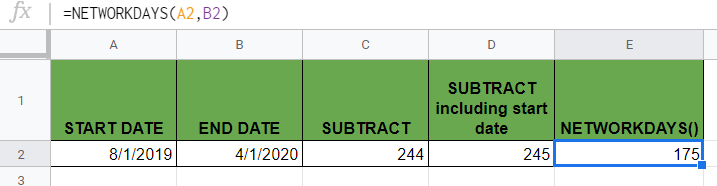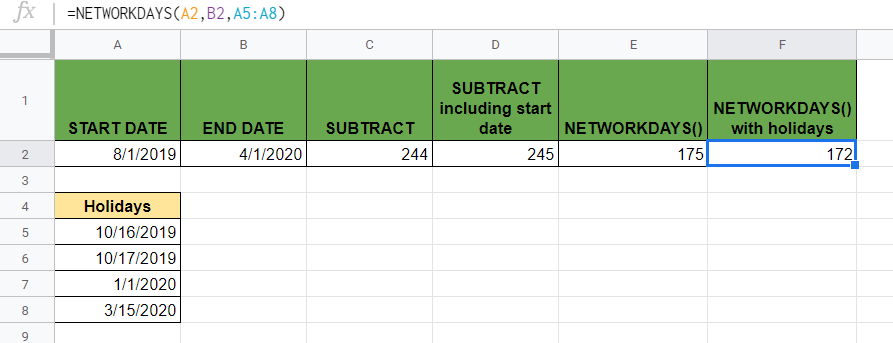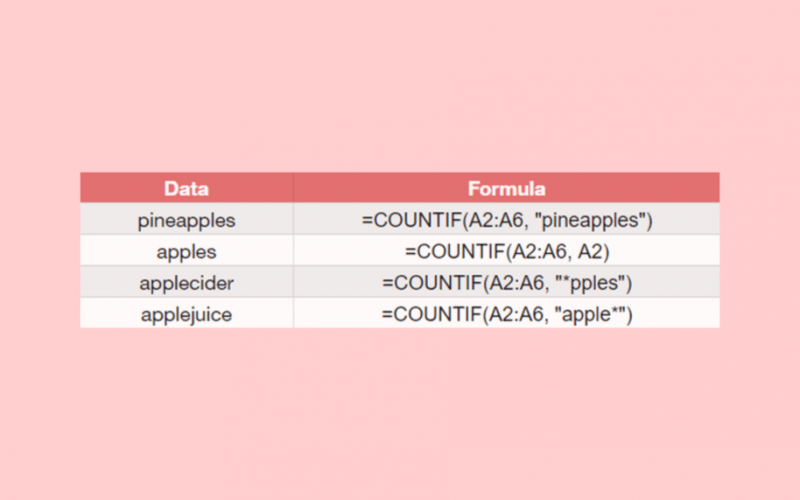# How To Calculate Days Between Dates In Google Sheets

To calculate days between dates in Google Sheets is easy by simply subtracting the dates. Also, we can use formulas to find out how many days, workdays, or months there are between two dates.

We almost always have to deal with dates in Google Sheets. Many times, we need to calculate how many days there are between two dates.

For example, how many days there are between August 1, 2019, and April 1, 2020. 📅

We have various possible ways to calculate this, but we will be sharing the three most straightforward ways which we found:

1. We can simply subtract the two dates to calculate the total number of days.
2. In other cases, we might want to calculate the number of workdays. In Google Sheets, we can do it with the `NETWORKDAYS` function.
3. Then, the `DATEDIF` function helps us calculate the total number of days, months, or years.

Let’s jump right in and take a look at each of them and their formulas so we can use them to calculate days between dates in Google Sheets.

## 1. Subtract the Dates to Calculate Days Between Dates

Say you have the start date and the end date, and you want to quickly know the total number of days between these two dates.The easiest way to calculate the number of days is to apply a simple subtraction operator in the formula.

The below formula will give you the total number of days between the two dates:

`=B2-A2`

Here we simply calculated the difference between the end date (B2) and the start date (A2).

As a result, the formula calculated that there are 244 days between August 1, 2019, and April 1, 2020.

This number of days is exclusive of the start date. In case we want to include the start date in the result, we need to add 1 to the result.

To get the number of days that include both the start date and the end date we should write the following formula:

`=B2-A2+1`This function outputs 245, so it includes the start day as well.

If the result you get is not a number but a date, you can convert the cell format to show a numeric value by selecting Format > Number > Number.

### ⚠️ A Note to Understand How Dates Work in Google Sheets

1. Google Sheets treats every Date and DateTime as numbers in the background. The date is stored as an integer, and the time is stored as a decimal fraction.
2. Since dates are just integer numbers, you can easily calculate the days between dates by subtracting the start date from the end date.

## 2. Use the NETWORKDAYS Function to Calculate Workdays Between Dates

While it’s easy to get the total number of days between two dates by subtracting them, in many cases, you need the difference of workdays.

The `NETWORKDAYS` function only counts the weekdays (from Monday to Friday), and it leaves weekends (Saturday and Sunday) out of the calculation.

The syntax of the function (the way we write it) is as follows:

`=NETWORKDAYS(start_date, end_date, [holidays])`

Let’s take a look at what this function and its variables mean:

• `=` the equal sign is how we start any function written in Google Sheets.
• `NETWORKDAYS` is our function. We need to add at least the required variables for it to work properly.
• `start_date` is the start date of the period from which we calculate the number of working days.
• `end_date` is the end date of the period from which we calculate the number of working days.
• `holidays` can be used as an optional variable. It is a range or array that consists of holiday dates. It’s an option we have to exclude additional holidays from the calculation.

### A Real Example Using the NETWORKDAYS Function

Say we want to calculate the number of working days between our two dates, August 1, 2019, and April 1, 2020.The following function does the job:

`=NETWORKDAYS(A2,B2)`

This function includes both the start date and end date when calculating the number of workdays.

Here’s what this example does:

• We selected cell D2 to write the formula in and started with the name of the function.
• Then, we added the `start_date` first, which is in cell A2.
• After that, we added the `end_date` which is in cell B2.
• In this example, we ignored the `holidays` variable, but it’s possible to add specific days that we want to exclude from the calculation.
• Finally, the function outputs 175, which is the total number of workdays excluding weekends between our start and end date.

Simple, right?

Go ahead and give it a shot! Using the link below you can make a copy of the example spreadsheet:

### Excluding Public Holidays from the Calculation

In many cases, we have some specific holidays that we want to exclude from the calculation of the workdays. For example, public holidays shouldn’t be counted as workdays.

The `NETWORKDAYS` function normally excludes weekends (Saturdays and Sundays) from the calculation. However, now we need to exclude even more days that we don’t want to count as workdays.

Take a look at the example below where we have some days in range A5:A8 that we don’t want to consider workdays. You can add any days that are your holidays.How can we tell the function that these days should not be counted?

Easy. We need to use the third variable of the `NETWORKDAYS` function to exclude some specific days from the calculation.

The function we write in cell E2 is as follows:

`=NETWORKDAYS(A2,B2,A5:A8)`

We simply add this range (A5:A8) of holidays as the `holidays` variable, and the function excludes them automatically.

As a result, we get 172, which is the number of workdays between the two dates, excluding the holidays defined by us.

## 3. Use the DATEDIF Function to Calculate Days Between Dates

The `DATEDIF` function helps us find the total days, months, or years between two dates. The name of the `DATEDIF` function stands for “date difference”.

The syntax of the function is as follows:

`=DATEDIF(start_date, end_date, unit)`

Let’s understand how to use this syntax and what each of these terms means:

• `=` the equal sign is how we start every function written in Google Sheets.
• `DATEDIF` is our function. We need to add the variables for it to work.
• `start_date` and `end_date` are the two dates. We will calculate the difference between these two dates. Be aware that here you should first write the start date, followed by the end date, unlike in a subtraction.
• `unit` variable indicates which measurement unit is the output displayed in. In simple cases, the unit for the function can be “D” (days), “M” (months), or “Y” (years). We should always write it between double quotation marks.

Now let’s begin using the `DATEDIF` function in our real examples.

### Use DATEDIF to Calculate Days Between Dates

Firstly, we will calculate the date difference in days.The following function calculates it:

`=DATEDIF(A2,B2,"D")`

As you can see, the function returned 244 as output, just like when we subtracted the dates.

### Use DATEDIF to Calculate Months and Years Between Dates

Similarly, we can calculate the number of whole months or years between two dates. We just have to change the unit variable to “M” or “Y”.

To calculate the number of entire months between the dates, we use the following formula:

`=DATEDIF(A2,B2,"M")`It returned 8, which is the number of months that have passed from August 1, 2019, and April 1, 2020.

To calculate the number of years, we simply change the last variable to “Y” as follows:

`=DATEDIF(A2,B2,"Y")`As a result, we got 0 since the two dates are less than one year apart from each other.

While the `DATEDIF` function has more options, it’s not always necessary to use it. If we just want to calculate the total number of days or workdays between two dates in Google Sheets, the first two solutions work perfectly too.That’s it, good job! You can now calculate days between dates together with the various other Google Sheets formulas to create even more powerful formulas. 🙂Our goal this year is to create lots of rich, bite-sized tutorials for Google Sheets users like you. If you liked this one, you'll love what we are working on! Readers receive ✨ early access ✨ to new content. There will be no spam and you can unsubscribe at any time.

##### You May Also Like## How to ROUND Numbers in Google Sheets

The ROUND function in Google Sheets is useful if you want to reduce a value by a specific…## How to Use ISBLANK Functions in Google Sheets

The ISBLANK function is useful if you want to find out whether a cell is empty. Let’s take…## How to Get Subscript and Superscript Values in Google Sheets

A few days back I was making some changes and adding data into a Google Sheet as part…## How to Insert Excel Table in Gmail with Borders

Occasionally you’ll want to insert an Excel table directly into your Gmail. Besides the fact that it looks…